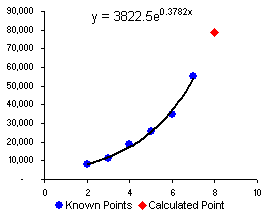Exponential growth can apply to population growth, economics, compound interest, bacteria growth, radiation, and many other natural phenomena. The growth of your savings account is a great example of exponential growth, though it may not be growing at the rate you really want it to. You can see this type of growth in action using a Savings Calculator or an Inflation Calculator. In these cases, it is fairly straight forward to just apply the FV() and PV() formulas, but that's not what this article is about.

This article explains how to calculate an exponential growth rate from a set of data using curving fitting and the LOGEST() function and how to use Excel's exponential growth function GROWTH() to make future predictions.

The formulas below show different ways of writing the exponential growth formula, where b is the initial value (or y-intercept) at x=0, r =m-1 is the growth rate, and x is the number of time intervals.

Exponential Growth Formula:
y = b*mx = b*eln(m)*x = b*(1+r)x

In business and finance, the most common exponential growth formula is the formula for compound interest. It is usually written using the symbols FV for future value, PV for present value, r for rate per period, and n for number of periods. Just equate FV=y, PV=b and n=x, and you've got the same formula as above.

Compound Interest:
FV = PV * (1+r)n

If you know the growth rate, it's easy to use the above equations to solve for future values. However, if the growth rate is not always the same or if you don't know the growth rate, you made need to use a curve-fit to create a exponential growth model that you can use for making predictions.

## Fitting an Exponential Growth Curve to Data

It is very easy to fit an exponential curve to a set of data in Excel. You can do that by just adding a exponential trendline to an x-y scatter plot. You can display the resulting equation in the chart as well, as shown in the figure below. The equation that is displayed will be in the form y=b*eln(m)x, but how do you get the growth rate from that?Fig 1. Example exponential growth curve.

The coefficients of the exponential growth model in figure 1 are shown below, along with the equation for calculating the growth rate:

m = EXP(0.3782)
b = 3822.5
r = EXP(0.3782) - 1

It's always a good idea to plot your data. If you don't plot the data, it's very difficult to know whether the exponential growth model is a very good fit. To create a more flexible spreadsheet (and avoid rounding errors) you may want to know how to calculate these coefficients using Excel formulas.

## Using LOGEST to Calculate Growth Rate

The LOGEST function uses the least squares method to fit an exponential curve to a set of known y-values and known x-values. It returns an array containing the m and b coefficients, so calculating the exponential growth rate is really quite simple.

m = INDEX(LOGEST(known_y's,known_x's),1,1)
b = INDEX(LOGEST(known_y's,known_x's),1,2)
r = INDEX(LOGEST(known_y's,known_x's),1,1) - 1

## Linearizing the Exponential Formula

To be complete, I must mention that you can also use the LINEST function or even the SLOPE and INTERCEPT functions to calculate the exponential growth function coefficients by first linearizing the formula (taking the natural log of both sides):

Linearized Exponential Growth Formula:
LN(y) = LN(m) * x + LN(b)

The linearized formula is just a line with slope = LN(m) and y-intercept = LN(b). If you were to plot LN(y) vs. x, you would get a straight line. You can also plot y vs. x and then change the y-axis to a logarithmic scale and you'll get a straight line. It is usually easier to visually judge goodness of fit for a line rather than an exponential curve.

m = EXP(INDEX(LINEST(LN(known_y's),known_x's),1,1))
m = EXP(SLOPE(LN(known_y's),known_x's))
b = EXP(INDEX(LINEST(LN(known_y's),known_x's),1,2))
b = EXP(INTERCEPT(LN(known_y's),known_x's))

## Using GROWTH to Make Predictions

After calculating the values for m and b, you can use the formula y=b*m^x to calculate a value for y given a time period x. However, you can also use the GROWTH function to do the exponential growth curve fit and find new values all in one step:

y = GROWTH(known_y's,known_x's,new_x)